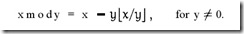# 原码, 反码, 补码 详解

## 二. 原码, 反码, 补码的基础概念和计算方法.

### 1. 原码

[+1] = 0000 0001

[-1] = 1000 0001

[1111 1111 , 0111 1111]

[-127 , 127]

### 2. 反码

[+1] =  = 

[-1] =  = 

### 3. 补码

[+1] =  =  = 

[-1] =  =  = 

## 三. 为何要使用原码, 反码和补码

[+1] =  =  = 

[-1] =  =  = 

1 - 1 = 1 + (-1) =  +  =  = -2

1 - 1 = 1 + (-1) = [0000 0001] + [1000 0001]= [0000 0001] + [1111 1110] = [1111 1111] = [1000 0000] = -0

1-1 = 1 + (-1) = [0000 0001] + [1000 0001] = [0000 0001] + [1111 1111] = [0000 0000]=[0000 0000]

(-1) + (-127) = [1000 0001] + [1111 1111] = [1111 1111] + [1000 0001] = [1000 0000]

-1-127的结果应该是-128, 在用补码运算的结果中, [1000 0000] 就是-128. 但是注意因为实际上是使用以前的-0的补码来表示-128, 所以-128并没有原码和反码表示.(对-128的补码表示[1000 0000]补算出来的原码是[0000 0000], 这是不正确的)

## 四 原码, 反码, 补码 再深入

1. 往回拨2个小时: 6 - 2 = 4

2. 往前拨10个小时: (6 + 10) mod 12 = 4

3. 往前拨10+12=22个小时: (6+22) mod 12 =4

2,3方法中的mod是指取模操作, 16 mod 12 =4 即用16除以12后的余数是4.

4 mod 12 = 4

16 mod 12 = 4

28 mod 12 = 4

### 负数取模x mod y = x - y L x / y J

x mod y等于 x 减去 y 乘上 x与y的商的下界.

-3 mod 2

= -3 - 2xL -3/2 J

= -3 - 2xL-1.5J

= -3 - 2x(-2)

= -3 + 4 = 1

(-2) mod 12 = 12-2=10

(-4) mod 12 = 12-4 = 8

(-5) mod 12 = 12 - 5 = 7

### 开始证明

(-2) mod 12 = 10

10 mod 12 = 10

-2与10是同余的.

(-4) mod 12 = 8

8 mod 12 = 8

-4与8是同余的.

a ≡ a (mod m)

(1)a ± c ≡ b ± d (mod m)

(2)a * c ≡ b * d (mod m)

7 ≡ 7 (mod 12)

(-2) ≡ 10 (mod 12)

7 -2 ≡ 7 + 10 (mod 12)

2-1=2+(-1) = [0000 0010] + [1000 0001]= [0000 0010] + [1111 1110]

(-1) mod 127 = 126

126 mod 127 = 126

(-1) ≡ 126 (mod 127)

2-1 ≡ 2+126 (mod 127)

2-1 与 2+126的余数结果是相同的! 而这个余数, 正式我们的期望的计算结果: 2-1=1

2-1=2+(-1) = [0000 0010] + [1000 0001] = [0000 0010] + [1111 1111]

[0111 1111] = 127

(-1) mod 128 = 127

127 mod 128 = 127

2-1 ≡ 2+127 (mod 128)

posted @ 2011-03-30 21:56  ziqiu.zhang  阅读(582615)  评论(130编辑  收藏  举报